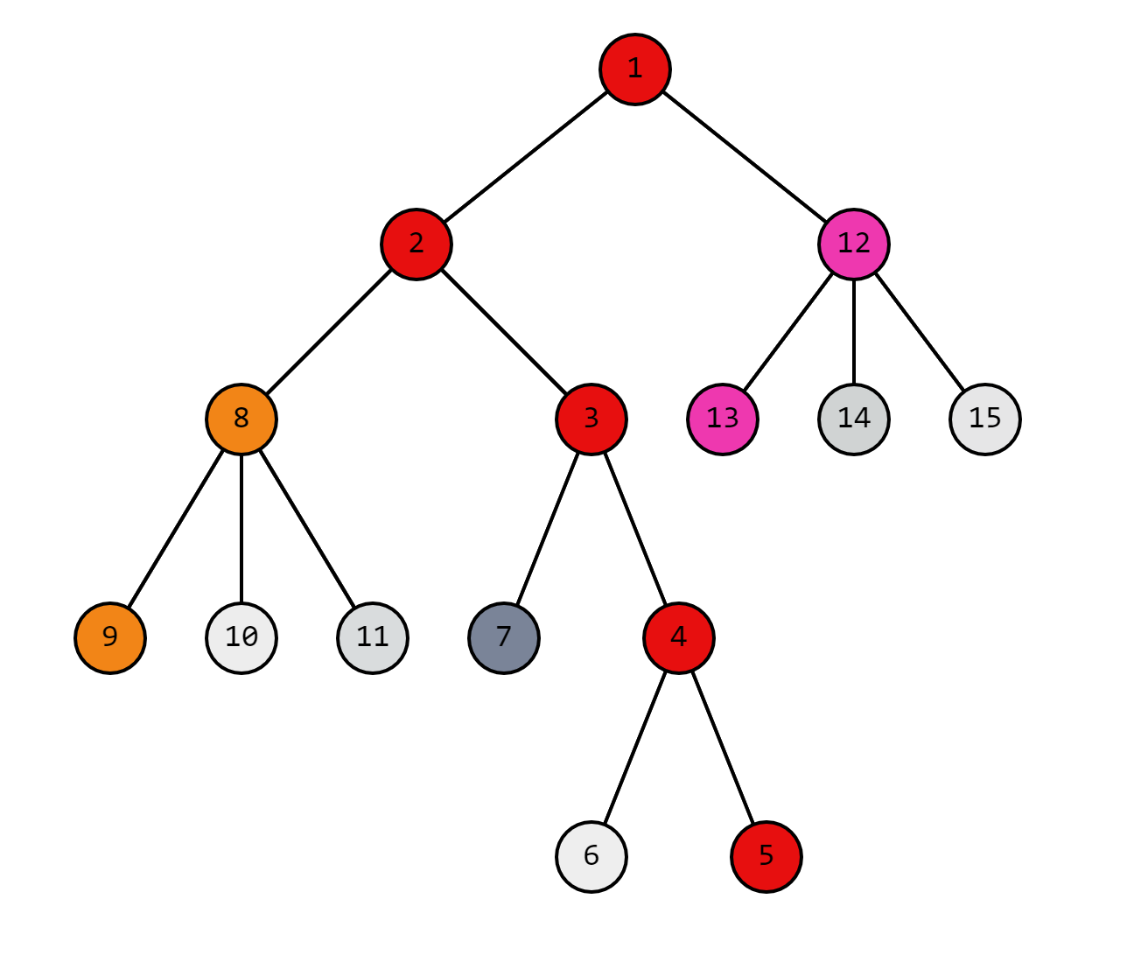# 树论## 树基础

### 无根树的等价定义

• 有 $n$ 个点，$n-1$ 条边的连通无向图
• 无向无环的连通图
• 任意两个结点之间有且仅有一条简单路径的无向图
• 任何边均为桥的连通图
• 没有环，且在任意不同两点间添加一条边之后所得图含唯一的一个环的图

### 有关树的定义

• 森林 (Forest) ：每一个连通块都是树的图（一棵树也是一个森林)
• 生成树 (Spanning Tree) ：一个连通无向图的生成子图，同时要求是树
• 结点的深度 (depth) ：到根结点的路径上的边数
• 树的高度 (height) ：所有结点深度的最大值
• 无根树的叶子 (leaf node) ：度数不超过 $1$ 的结点
• 有根树的叶子 (leaf node) ：没有子结点的结点

### 有根树的定义

• 父亲 **(parent node)**：对于除根以外的每个结点，定义为从该结点到根路径上的第二个结点。 根结点没有父结点。
• 祖先 **(ancestor)**：一个结点到根结点的路径上，除了它本身外的结点。 根结点的祖先集合为空。
• 子结点 **(child node)**：如果 $u$ 是 $v$ 的父亲，那么 $v$ 是 $u$ 的子结点。
• 兄弟 **(sibling)**：同一个父亲的多个子结点互为兄弟。
• 后代 **(descendant)**：如果 $u$ 是 $v$ 的祖先，那么 $v$ 是 $u$ 的后代。
• 子树 **(subtree)**：删掉与父亲相连的边后，该结点所在的子图（所有到根结点路径上经过 $u$ 的结点形成子图)。### 特殊的树

• 链 **(chain)**：满足与任一结点相连的边不超过 $2$ 条的树称为链。• 菊花 / 星星 **(star)**：满足存在 $u$ 使得所有除 $u$ 以外结点均与 $u$ 相连的树称为菊花。#### 例题

1. 给定路径的端点 $u,v$，我们可以用 $n$ 次询问找到路径上所有点
2. 给定路径的端点 $u,v$，我们可以询问 $a$，$a$ 到 $v$ 的路径是否有 $u$，不断调整，可以用 $2n$ 次询问将 $u,v$ 转移到两个不同叶子。
3. 对于一条叶子和叶子之间路径，如果路径长度为 $2\times height-1$，那么这条路径必定经过根，对于根结点，恰好有 $dep-2$ 个结点分别在到两个叶子中间，询问次数 $\log^2 n$。

## dfs序

$dfs$ 序是在深度优先遍历的过程中，进入每个结点的时间戳，即记录第几个访问到的结点是什么。$dfs$ 序有一个很好的性质是一棵子树内的所有结点是 $dfs$ 序上的一段连续区间。

### 经典问题

1. 将 $u$ 的子树内所有点的权值加 $x$
2. 询问 $u$ 的子树内所有点的权值和

$1 \le n,q \le 10^5$

### 例题3

1. 所有深度为 $l$ 的点权值加上 $x$
2. 询问子树的权值和

1. 树状数组维护，暴力修改所有深度为 $l$ 的单点，时间复杂度：$O(n \log n)$
2. 在深度 $l$ 上打标记，预处理 $dfs$ 序深度为 $d$ 的个数的前缀和，时间和空间复杂度：$O(n^2)$，回答询问的时间复杂度为 $O(n)$。

## 最近公共祖先

LCA 是树上的一个经典问题，也是解决很多树上问题的重要理论依据和解决工具。

### 朴素

1. 将一个点往父亲跳到根，记录一下经过了哪些点，在把另外一个点往上跳，第一个相遇的点就是最近公共祖先。显然一条链情况下，单次查询复杂度 $O(n)$。
2. 先调整深度大的结点，令他们深度相同，因此他们到 $LCA$ 的距离相同，所以可以两个点一起往上跳，第一个相遇的点就是最近公共祖先，单次查询复杂度 $O(n)$。### 倍增

$$fa[x][i]=fa[fa[x][i-1]][i-1]$$

### 应用

#### 路径长度

$LCA$ 的一个重要应用就是可以计算树上两点之间路径长度。##### 例题1

$n$ 个点 $n$ 条边的无向连通图，而一棵 $n$ 个点的树有 $n-1$ 条边，现在是在一棵树上加了一条环边。

#### 树上差分

$LCA$ 可以用于树上打标记差分。

##### 例题1

1. 一条树边不在任何一个环内，切断这条边，图已经不连通，再随意切一条环边即可。
2. 一条树边仅在一个环内，切断这条边和对应的环边，图不连通。
3. 一条树边在多个环内，那么必须把这些环边全部切断才能使得图不连通。

#### 例题

（怎么看这个题都非常的神奇，又是换根又是要弄一棵虚树出来计数。

$$S(n,m)=S(n-1,m-1)+m\cdot S(n-1,m)$$

$$dp[i][j]=dp[i-1][j-1]+(j-h[i])dp[i-1][j](h[i]<j\le m)$$

### 树链剖分

#### 引入

1. 修改 单个结点 / 树上两点之间的路径 / 一个结点的子树上 的所有点的值。
2. 查询 单个结点 / 树上两点之间的路径 / 一个结点的子树上 结点的值的 **和(其他支持线段树区间合并的信息)**。

#### 路径剖分$dfs$ 过程中，对于第一个儿子，我们将他放入父亲所处的这条链内，具体来说就是设置他的 top 值和父亲相同即可。然后，对于其他的儿子，我们设置他的 top 值为其自身，表示从这个点开始新开了一条链。

#### 轻重链剖分##### 定义

size[u] 表示以 $u$ 为根的子树大小，即子树内结点个数。

son[u] 表示 $u$ 的所有儿子中，对应子树结点数最多的那个儿子，即重儿子，其余儿子均为轻儿子。

##### 性质
1. 假设在以 $u$ 为根的子树中，$v$ 是 $u$ 的轻儿子，有 $size[v] \le {size[u] \over 2}$。

证明：如果 $size[v] > {size[u] \over 2}$，那么 $v$ 的大小将大于 $u$ 的其它所有子树大小之和，这与 $v$ 是 $u$ 的轻儿子矛盾。

2. $u$ 到根路径上最多有 $O(\log n)$ 条重链或轻边。

证明：从根结点到一个结点的路径上，每次经过一条轻边，子树大小将会至少缩小一半，因此这条路径上至多有 $\log n$ 条轻边。再考虑重链，因为重链一定是在路径的开始，结束位置或者两条轻边之间，所以重链的数量级是和轻边相同，因此 $u$ 到根的路径上最多只有 $O(\log n)$ 条重链或轻边。1. 加边和删边
2. 树上路径操作
3. 子树操作

### 转化为 RMQ 问题1 2 3 4 5 6 7 8 9 10 11 12 13 14 15 16 17 18 19
1 2 5 2 6 9 6 10 6 2 1 3 1 4 7 8 7 4 1

1. 通过 $dfs$ 序将树上 $LCA$ 问题转化为序列 RMQ 问题
2. 单调栈将序列转化为笛卡尔树
3. 在笛卡尔树上求欧拉序，转化为 $\pm 1$ RMQ 问题
4. 对新序列分块，做分块 ST 表，块内通过二进制状压 DP 维护

### Tarjan

Tarjan 算法核心是进行一次深度优先搜索，类似于转化为 RMQ 问题的思路，$u$ 和 $v$ 的最近公共祖先就是从 $u$ 遍历到 $v$ 这个过程中，深度最小的点就是 $LCA$。于是，我们将每个询问放到对应的结点上，处理一个结点上的询问时，就是判断另外一个点是否访问过，如果另外一个点访问过的话，我们要找出它是回溯到哪个时才遍历到这个点，这个可以用并查集进行维护。

## 树上启发式合并

Codeforces 原文链接：dsu on tree

### 例题

$n$ 个选手，一开始每个人被随机发了一张石头剪刀布卡片，显然有 $3^n$ 种分配方式。

1. 1 x y：撤去座位 $y$ ，$y$ 上的人 $b$ 和坐在 $x$ 上的人 $a$ 石头剪刀布，如果 $b$ 赢了则 $a$ 被淘汰，$b$ 坐在 $x$ 上，否则 $b$ 被淘汰（保证数据合法）。
2. 2 x：进行了之前的一类事件后，有多少种分配方式使得第 $x$ 个选手没被淘汰。

## 树哈希

$$f_{now}=1+\sum f_{son_{now,i}} \times prime(size_{son_{now,i}})$$

$size_{x}$ 表示以结点 $x$ 为根的子树大小。

$son_{x,i}$ 表示 $x$ 所有子结点之一（不用排序）。

$prime(i)$ 表示第 $i$ 个质数。

## 问题

### 例题1

$dfs$ 到某一个结点时，考虑这个结点所有儿子的权值，这些权值有正有负，所有负的权值的和，也就是这个子树总共还有多个点没有被覆盖。

### 例题5

$$dp[u]=0 \ dp[u]=c_i \ dp[u]=c_i-d_i$$

$$dp[u][i+j]=min(dp[u][i]+dp[v][j]) \ dp[u][i+j]=min(dp[u][i]+dp[v][j]) \ dp[u][i+j]=min(dp[u][i]+dp[v][j])$$

$$sum=1+size(v_1)+size(v_2)+\dots+size(v_k)$$

2019 年江苏省赛 I Neko and tree

2018 年宁夏邀请赛 G Factories

### 例题6

$$\sum_{X\subseteq V,X \neq \varnothing} (f(X))^k$$

$$(f(X))^k=\sum_{i=0}^{k}S(k,i)i!{f(X) \choose i}$$

$$\sum_{X\subseteq V,X \neq \varnothing} {f(X) \choose i}$$

$$\sum_{X\subseteq V,X \neq \varnothing} \sum_{e_j \in E’,1\le j \le i} [e_1,e_2,\dots,e_i \in E_X]$$

$$\sum_{e_j \in E’,1\le j \le i} \sum_{X\subseteq V,X \neq \varnothing} [e_1,e_2,\dots,e_i \in E_X]$$

1. $u$ 到子树的边单独构成边集。

2. $u$ 到子树的边与这棵子树的边集合并。

3. $u$ 的子树的边集之间合并，子树的边集包含前两种情况。

## 其他科技 / Trick

### 基环树

$n$ 个点 $n$ 条边的联通图是一棵基环树，边由 $n-1$ 条树边加上一条环边组成。

#### 思路

• 拆出一条环边，剩余当成树做。

• 抠出整个环，环上每个点挂了一棵子树，先做 Tree dp，在环上做序列 dp。

## 最后

“树论” 介绍了在一般树上的算法和问题。

$dfs$ 序的性质和应用，使用 $dfs$ 序将树上问题放到序列上处理，利用 $dfs$ 遍历树过程中的特性处理问题。介绍了计算最近公共祖先的朴素算法，倍增算法，转化为 RMQ 问题和离线的 Tarjan 算法，以及处理树上路径操作问题的轻重链链剖分算法，并应用最近公共祖先和差分的思想，高效处理了几个树上路径长度和打标记的问题。最后，树分治结构可以解决一类在无根树上的路径统计问题，树上启发式合并可以解决一类在静态树上子树信息查询问题。